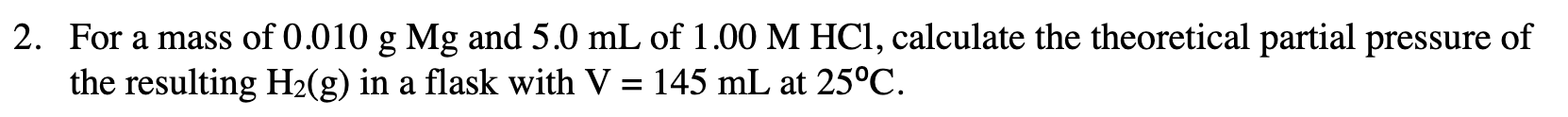# 2. For a mass of 0.010 g Mg and 5.0 mL of 1.00 M HCl, calculate the theoretical partial pressure of the resulting H2(g) in a flask with V = 145 mL at 25°C

Questionhelp_outlineImage Transcriptionclose2. For a mass of 0.010 g Mg and 5.0 mL of 1.00 M HCl, calculate the theoretical partial pressure of the resulting H2(g) in a flask with V = 145 mL at 25°C fullscreen

### Want to see the step-by-step answer?